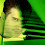## Thursday, September 26, 2013

### Geometry Problem 928: Triangle, Midpoint, Median, Cevian, Concurrency, Area

Geometry Problem
Level: Mathematics Education, High School, Honors Geometry, College.

Click the figure below to see the complete problem 928.1.Denote the area of ΔABC by S(ABC).

Obviously, S(AME)=S(CME). i.e. S5=S6.

By Ceva's theorem, BF/AF=BD/CD. i.e. FD//AC.
Thus, S(FAC)=S(DAC), which implies S1=S4.

Lastly, S(BAM)=S(BCM), which implies S1=S4.

Hence, S1+S3+S5 = S2+S4+S6 = S/2.

2.By affine transformation to any isosceles triangle, all the properties in the problem is preserved.
After the transformation,
S1=S4 ; S2=S3 ; S5=S6
As affine preserved area ratio, the proof is completed.

3.http://www.mathematica.gr/forum/viewtopic.php?f=22&t=40193&p=187258#p187258

4.S6=S5 => h1=h2 (h1=AA', h2=CC' alt)
=> h3=h4 (h3=FF', h4=DD') because Right tr AA'B~ tr FF'B and BCC'~BFF'
=> S2=S3 => S1=S4